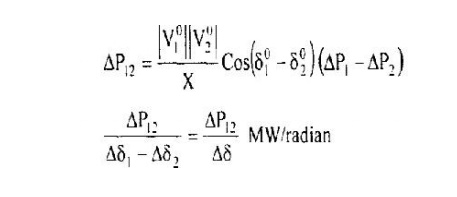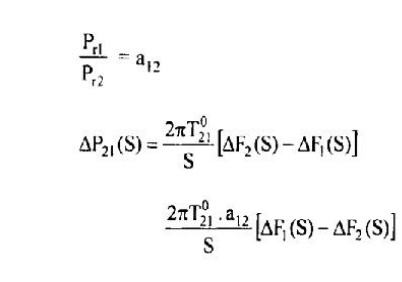Home | | Power System Operation and Control | Two Area Systems - Tie-Line Power Model

# Two Area Systems - Tie-Line Power Model

Consider two inter connected areas as shown in figure operating at the same frequency fl while a power Power flow from area I to area 2

TWO AREA SYSTEMS - TIE-LINE POWER MODELConsider two inter connected areas as shown in figure operating at the same frequency fl while a power Power flow from area I to area 2

let V1 and V 2 be the voltage magnitudes

δ1, δ 2 voltage phase angles at the two ends of the tie-line While P flows from area I to area 2 then,Where X is the reactance of the line. If the angles change by f1o1, and f102 due to load changes in areas I and 2 respectively. Then, the tie-line power changes byStudy Material, Lecturing Notes, Assignment, Reference, Wiki description explanation, brief detail
Power System Operation and Control : Real Power Frequency Control : Two Area Systems - Tie-Line Power Model |